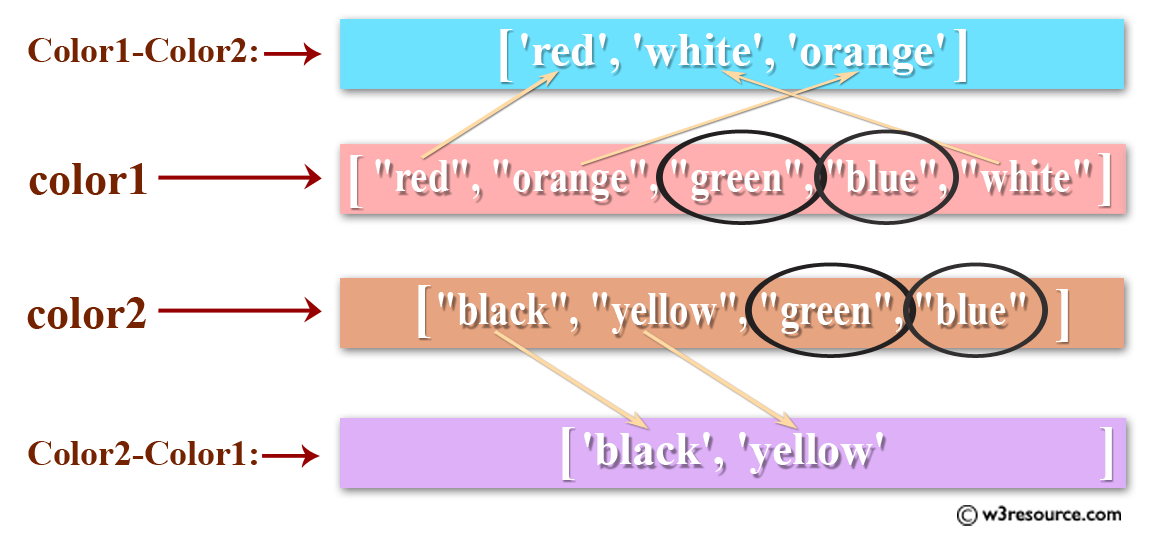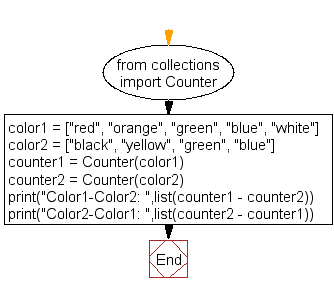﻿ Python: Compute the difference between two lists - w3resource# Python: Compute the difference between two lists

## Python List: Exercise - 52 with Solution

Write a Python program to compute the difference between two lists.Sample Solution :-

Python Code :

``````from collections import Counter
color1 = ["red", "orange", "green", "blue", "white"]
color2 = ["black", "yellow", "green", "blue"]
counter1 = Counter(color1)
counter2 = Counter(color2)
print("Color1-Color2: ",list(counter1 - counter2))
print("Color2-Color1: ",list(counter2 - counter1))
```
```

Sample Output:

```Color1-Color2:  ['red', 'white', 'orange']
Color2-Color1:  ['black', 'yellow']
```

Flowchart:## Visualize Python code execution:

The following tool visualize what the computer is doing step-by-step as it executes the said program:

Python Code Editor:

Have another way to solve this solution? Contribute your code (and comments) through Disqus.

What is the difficulty level of this exercise?

Test your Python skills with w3resource's quiz

﻿

## Python: Tips of the Day

Floor Division:

When we speak of division we normally mean (/) float division operator, this will give a precise result in float format with decimals.

For a rounded integer result there is (//) floor division operator in Python. Floor division will only give integer results that are round numbers.

```print(1000 // 300)
print(1000 / 300)```

Output:

```3
3.3333333333333335```